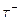Chapter 33, Problem 20PE

Chapter
Section
Textbook Problem

(a) What is the uncertainty in the energy released in the decay of adue to its short lifetime?(b) Is the uncertainty in 1his energy greater than or lees than the uncertainty in the mass of the tau neutrino? Discuss the source of the uncertainty.

To determine

(a)

The uncertainty in the energy released in the decay of a τ particle due to its short life time.

Explanation

Given:

τ particle is having short life time.

Formula used:

The uncertainty principle for energy and time is given by, ΔE.Δt=h4π

Where, ΔE uncertainty in the measurement of energy

Δt uncertainty in the measurement of time

Calculation:

Life time of τ particle =2.91×1013 s

Therefore, uncertainty in the energy released, ΔE=h4πΔt

=6.6×10344×3

To determine

(b)

Whether the uncertainty in the energy released in the decay of τ particle is greater than

or less than the uncertainty in the mass of tau neutrino.

Still sussing out bartleby?

Check out a sample textbook solution.

See a sample solution

The Solution to Your Study Problems

Bartleby provides explanations to thousands of textbook problems written by our experts, many with advanced degrees!

Get Started